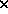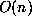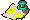One of the interesting morals from theoretical computer science is that many familiar algorithms are far from optimal. In order to be more precise we introduce the notion of bit complexity. Bit complexity counts the number of single operations required to complete an algorithm. The single-digit operations are +, -,. (We could, if we wished, introduce storage and logical comparison into the count. This, however, doesn't affect the order of growth of the algorithms in which we are interested.) This is a good measure of time on a serial machine. Thus, addition of two n-digit integers by the usual method has bit complexity, straightforward uniqueness considerations show this to be asymptotically best possible.Annotation Form Interface```